# Math in Focus Grade 6 Chapter 8 Lesson 8.4 Answer Key Real-World Problems: Equations and Inequalities

Practice the problems of Math in Focus Grade 6 Workbook Answer Key Chapter 8 Lesson 8.4 Real-World Problems: Equations and Inequalities to score better marks in the exam.

## Math in Focus Grade 6 Course 1 B Chapter 8 Lesson 8.4 Answer Key Real-World Problems: Equations and Inequalities

### Math in Focus Grade 6 Chapter 8 Lesson 8.4 Guided Practice Answer Key

Complete.

Question 1.
On Monday, Wendy had some leaves in a collection she was making for biology class. After she collected 23 more leaves on Tuesday, she had 41 leaves. Find the number of leaves Wendy had on Monday.
Let r represent the number of leaves Wendy had on Monday.
r + 23 =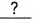r + 23 –=r =Wendy hadleaves on Monday.
Wendy had 18 leaves on Monday,

Explanation:
Given on Monday, Wendy had some leaves in a collection she was making for biology class. After she collected 23 more leaves on Tuesday, she had 41 leaves. The number of leaves Wendy had on Monday. Let r represent the number of leaves Wendy had on Monday so r + 23 = 41, r = 41 – 23 = 18 leaves.

Write an algebraic equation for each problem. Then solve.

Question 2.
Carlos thinks of a number. When he adds 17 to it, the result is 45. What is the number that Carlos thought of?
The number is 28,

Explanation:
Given Carlos thinks of a number. When he adds 17 to it, the result is 45. Let the number that Carlos thought of is x so x + 17 = 45, therefore x = 45 – 17 = 28.

Question 3.
Sylvia bought some blouses and T-shirts. She paid a total of $63. The T-shirts cost$29. How much did the blouses cost?
Blouses cost $34, Explanation: Given Sylvia bought some blouses and T-shirts. She paid a total of$63. The T-shirts cost $29. Let the blouses be b so it is$29 + b = $63, So b =$63 – $29 =$34.

Question 4.
Felicia used 153 yellow beads and some green beads for her art project. She used 9 times as many yellow beads as green beads. How many green beads did she use for the project?

Explanation:
Given Felicia used 153 yellow beads and some green beads for her art project. She used 9 times as many yellow beads as green beads. Let g be green beads did she use for the project so we have green beads as 9 X 153 = 1,377 beads.

Question 5.
Ivan had saved some quarters. He spent 50 quarters, which was $$\frac{2}{5}$$ of the quarters he started out with. How many quarters did he start out with?
Quarters did Ivan start out with is 125,

Explanation:
Given Ivan had saved some quarters. He spent 50 quarters, which was $$\frac{2}{5}$$ of the quarters he started out with. Let x quarters did he starts so x X $$\frac{2}{5}$$ = 50, x = 50 X $$\frac{5}{2}$$ =
125.

Complete.

Question 6.
The figure shows a speed limit sign on a highway.
a) Let x represent the speed in miles per hour.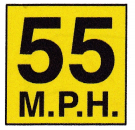Write an inequality to represent the situation.
The inequality is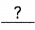.
The inequality is x ≤ 55,

Expanation:
Given fiqure shows a speed limit sign on highway, Let x represent the speed in miles per hour so an inequality to represent the situation is x ≤ 55.
b) Give the maximum legal driving speed on the highway. The maximum legal driving speed ismiles per hour.
The maximum legal driving speed is 55 miles per hour,

Explanation:
Given fiqure shows a speed limit sign on highway, So the maximum legal driving speed is 55 miles per hour.

Solve.

Question 7.
More than 35 guests came to Katrina’s birthday party last Sunday.

a) Write an inequality to represent the number of guests who turned up for the birthday party.
x>35,

Explanation:
Given more than 35 guests came to Katrina’s birthday party last Sunday, Let x represent the number of guests turned up for the birthday party, so the inequality to represent the number of guests who turned up for the birthday party are  x > 35.

b) What is the least possible number of guests who could have come to the party?
35,

Explanation:
Given more than 35 guests came to Katrina’s birthday party last Sunday, So the least possible number of guests who could have come to the party are 35.

Question 8.
In Mr. Boyle’s class, the students are required to summarize a passage in less than 50 words.
a) Write an inequality to represent the number of words that the students can use to summarize the passage.
x < 50,

Explanation:
Given in Boyle’s class, the students are required to summarize a passage in less than 50 words. So the inequality to represent the number of words that the students can use to summarize the passage , let it be x so x < 50.

b) What is the maximum number of words that a student can use?
50,

Explanation:
Given in Boyle’s class, the students are required to summarize a passage in less than 50 words. So the maximum number of words that a student can use are 50.

Question 9.
A cargo elevator has a load limit of 240 tons.
a) Write an inequality to represent the load limit of the cargo elevator.
x ≤ 240,

Explanation:
Given a cargo elevator has a load limit of 240 tons. Let load limit be x so an inequality to represent the load limit of the cargo elevator is x ≤ 240.

b) What is the greatest possible load the cargo elevator can carry?
240 tons,

Explanation:
Given a cargo elevator has a load limit of 240 tons. So the greatest possible load the cargo elevator can carry is 240 tons.

Question 10.
To get a discount coupon at a bookstore, you need to spend at least $50 at the store. a) Write an inequality to represent the amount of money that you must spend in order to get a discount coupon. Answer: x ≥ 50, Explanation: Given to get a discount coupon at a bookstore we need to spend at least$50 at the store. Let amount spend be x so an inequality to represent the amount of money that must be spent in order to get a discount coupon is x ≥ 50.

b) Andrea has spent $45 at the store, and her friend Alex has spent$55. Which person can get a discount coupon?
Alex will get a discount coupon,

Explanation:
Given to get a discount coupon at a bookstore we need to spend at least $50 at the store as Andrea has spent$45 which is less than $50 will not get the discount and Alex has spent$55 which is more than $50 so Alex will get a discount coupon. ### Math in Focus Course 1B Practice 8.4 Answer Key Write and solve an algebraic equation for each problem. Show your work. Question 1. Damien thinks of a number. When he adds 32 to it, the sum is 97. What is the number that Damien thought of? Answer: 65, Explanation: Given Damien thinks of a number. Let it be x when he adds 32 to it, the sum is 97. So the number that Damien thought of is x + 32 = 97, x = 97 -32 = 65. Question 2. A baker made some bagels in the morning. After selling 85 bagels, there were 64 left. How many bagels did the baker make in the morning? Answer:149 bagels, Explanation: Given a baker made some bagels in the morning. Let it be x after selling 85 bagels there were 64 left. So many bagels did the baker make in the morning are x – 85 bagels= 64 bagels, therefore x = 64 bagels + 85 bagels = 149 bagels. Question 3. Claudia can text 3 times as fast as Fiona. Claudia can text 78 words per minute. How many words per minute can Fiona text? Answer: 26 words per minute, Explanation: Given Claudia can text 3 times as fast as Fiona. Claudia can text 78 words per minute means Fiona = Claudia/3 so many words per minute can Fiona text are 78/3 = 26 words per minute. Question 4. Eric spent $$\frac{2}{5}$$ of his allowance on a jacket. The jacket cost him$12. How much was his allowance?
Allowance is $30, Explanation: Given Eric spent $$\frac{2}{5}$$ of his allowance on a jacket. The jacket cost him$12. Let A be the allowance so much was his allowance is A = $12 X $$\frac{5}{2}$$ = $$\frac{12 X 5}{2}$$ =$30.

Write and solve an algebraic inequality for each problem.

Question 5.
In a science competition, students have to score more than 40 points in order to move on to the next round.
a) Write an inequality to represent this situation. Use a number line to represent the inequality.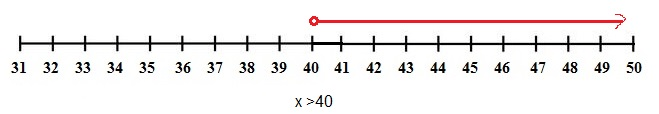Explanation:
Given in a science competition students have to score more than 40 points in order to move on to the next round,
So an inequality to represent this situation is x > 40. Used a number line to represent the inequality as shown above.

b) What is the least number of points a student needs to score in order to move on to the next round? Only whole numbers of points are awarded to students.
41,

Explanation:
As given in a science competition students have to score more than 40 points in order to move on to the next round,
So the least number of points a student needs to score in order to move on to the next round if only whole numbers of points are awarded to students is 41.

Question 6.
A stadium has a seating capacity of 65,000 spectators.
a) What is the maximum number of spectators the stadium can hold?
65,000 spectators,

Explanation:
Given a stadium has a seating capacity of 65,000 spectators. So the maximum number of spectators the stadium can hold is 65,000 spectators.

b) Write an inequality to represent this situation. Then use a number line to represent the inequality.
x ≤ 65,000,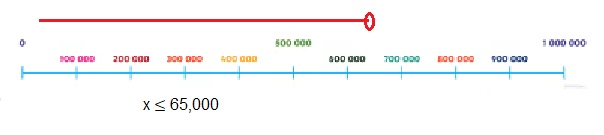Explanation:
Given a stadium has a seating capacity of 65,000 spectators. Inequality to represent this situation is x ≤ 65,000. Then used a number line to represent the inequality as shown above.

Write and solve an algebraic equation or inequality for each problem.

Question 7.
A bicycle store sells $$\frac{4}{7}$$ of the mountain bikes in the store. Then only 24 mountain bikes are left. How many mountain bikes were there originally?
42 mountain bikes,

Explanation:
Given a bicycle store sells $$\frac{4}{7}$$ of the mountain bikes in the store. Then only 24 mountain bikes are left. Let x be mountain bikes were there originally so x X $$\frac{4}{7}$$ = 24 mountain bikes, x = 24 mountain bikes X $$\frac{7}{4}$$ = $$\frac{24 X 7}{4}$$ mountain bikes = 6 X 7 = 42 mountain bikes.

Question 8.
Mabel has a total of 54 decorative beads. Some are black and some are white. The ratio of the number of black beads to the number of white beads is 7 : 2. How many more black beads than white beads are there?

Explanation:

Question 9.
Gary has a collection of comic books. After selling 70% of his comic books, he has 42 comic books left. How many comic books did he start with?

Explanation:
Given Gary has a collection of comic books. After selling 70% of his comic books, he has 42 comic books left. So many comic books did he start with be x is x X 70% = 42 comic books,  x = 42 X 100/70 = 60 comic books.

Question 10.
There are 30 students in the gym. If there are at least 16 girls, write an inequality to represent the number of boys in the gym.
x ≤ 14,

Explanation:
Given there are 30 students in the gym. If there are at least 16 girls, So an inequality to represent the number of boys in the gym let it be x as number of boys + number of girls = 30 so number of boys are 30 – 16 = 14 therefore it is less than or equal to 14 so x ≤ 14.

Question 11.
The marbles in a box are repackaged in equal numbers into 6 smaller bags. If each bag has more than 8 marbles, what is the least possible number of marbles that could have been in the box?
48 marbles,

Explanation:
Given the marbles in a box are repackaged in equal numbers into 6 smaller bags. If each bag has more than 8 marbles, let x be the least possible number of marbles that could have been in the box so it is x = 6 X 8 = 48 marbles.

Question 12.
Mr. Edwards is now 3 times as old as his daughter. In 15 years’ time, the sum of their ages will be 86.
a) Find their ages now.
Father 42 years, Daughter 14 years,

EXplanation:
Given Mr. Edwards is now 3 times as old as his daughter. In 15 years’ time, the sum of their ages will be 86. Let the age of the daughter be x so 3x + 15 + x + 15 = 86, 4x + 30 = 86, 4x = 86 – 30 = 56, So x = 56/4 = 14 years. So fathers age is 3 X 14 years = 42 years.

b) How old was Mr. Edwards when his daughter was born?
Mr.Edwards was 28 years when his daughter was born,

Explanation:
If the ages of father is 42 years and daughter age is 14 years then Mr.Edwards age when his daughter was born is 42 years – 14 years = 28 years.

Question 13.
In a competition, each school is allowed to send a team with at least 5 members, but not more than 8 members. 12 schools participated in the competition.
a) Find the least possible number of participants in the competition.
60 participants,

Explanation:
Given in a competition, each school is allowed to send a team with at least 5 members. 12 schools participated in the competition. Let x be the least possible number of participants in the competition so x = 12 X 5 = 60 participants.

b) Find the greatest possible number of participants in the competition.